# 17.2: Enolization of Aldehydes and Ketones

$$\newcommand{\vecs}{\overset { \rightharpoonup} {\mathbf{#1}} }$$ $$\newcommand{\vecd}{\overset{-\!-\!\rightharpoonup}{\vphantom{a}\smash {#1}}}$$$$\newcommand{\id}{\mathrm{id}}$$ $$\newcommand{\Span}{\mathrm{span}}$$ $$\newcommand{\kernel}{\mathrm{null}\,}$$ $$\newcommand{\range}{\mathrm{range}\,}$$ $$\newcommand{\RealPart}{\mathrm{Re}}$$ $$\newcommand{\ImaginaryPart}{\mathrm{Im}}$$ $$\newcommand{\Argument}{\mathrm{Arg}}$$ $$\newcommand{\norm}{\| #1 \|}$$ $$\newcommand{\inner}{\langle #1, #2 \rangle}$$ $$\newcommand{\Span}{\mathrm{span}}$$ $$\newcommand{\id}{\mathrm{id}}$$ $$\newcommand{\Span}{\mathrm{span}}$$ $$\newcommand{\kernel}{\mathrm{null}\,}$$ $$\newcommand{\range}{\mathrm{range}\,}$$ $$\newcommand{\RealPart}{\mathrm{Re}}$$ $$\newcommand{\ImaginaryPart}{\mathrm{Im}}$$ $$\newcommand{\Argument}{\mathrm{Arg}}$$ $$\newcommand{\norm}{\| #1 \|}$$ $$\newcommand{\inner}{\langle #1, #2 \rangle}$$ $$\newcommand{\Span}{\mathrm{span}}$$$$\newcommand{\AA}{\unicode[.8,0]{x212B}}$$

Transformation of a carbonyl compound to an enol at a useful rate normally requires either a basic catalyst or an acidic catalyst and, of course, at least one hydrogen on the $$\alpha$$ carbon. The features of each type of catalysis follow.

## Enolization in Basic Solution. $$\ce{C-H}$$ Acidity of Carbonyl Compounds

With a basic catalyst such as hydroxide ion, the first step in enolization is removal of a proton from the $$\alpha$$ position to give the enolate anion $$1$$:Normally, $$\ce{C-H}$$ bonds are highly resistant to attack by basic reagents, but removal of a proton alpha to a carbonyl group results in the formation of a considerably stabilized anion with a substantial proportion of the negative charge on oxygen, as represented by the valence-bond structure $$1a$$. Carbonyl compounds such as 2-propanone therefore are weak acids, only slightly weaker than alcohols (compare the p$$K_\text{a}$$ values for some representative compounds in Table 17-1).$$^1$$

Table 17-1: $$\ce{C-H}$$ and $$\ce{O-H}$$ Acidities of Some Representative Compounds$$^a$$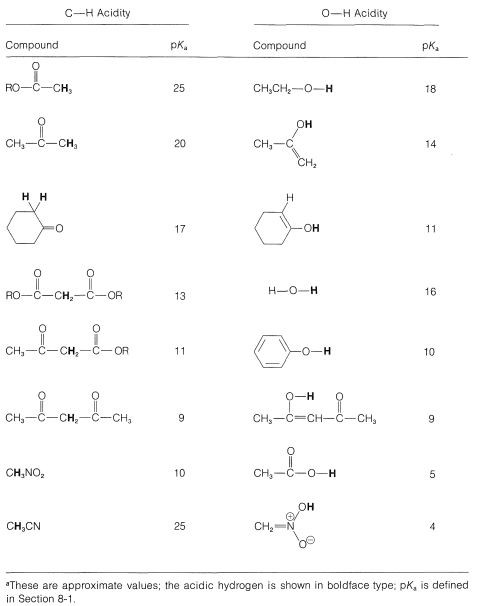Two carbonyl groups greatly increase the acidity. For example, 2,4-pentanedione (acetylacetone, $$2$$) has a p$$K_\text{a} \cong$$ 9, which is comparable to the $$\ce{O-H}$$ acidity of phenols (see Table 17-1). The reason is that the enolate anion $$3$$ has the charge largely shared by the two oxygen atoms (cf. $$3b$$ and $$3c$$). As a result, the enolate anion $$3$$ is stabilized more with respect to the ketone than the enolate anion from 2-propanone is stabilized relative to 2-propanone:## Enol Formation from Enolate Anions

You will notice from Structures $$1a$$ and $$1b$$ that because the negative charge of the enolate anion is distributed on both oxygen and carbon, the ion can, in principle, combine with a proton at either site. If the enolate ion adds a proton to oxygen, the enol is formed; if it adds a proton to carbon, the ketone is formed: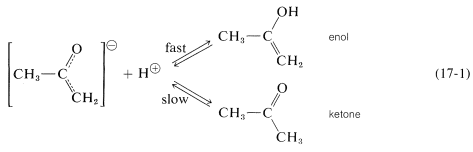Ions of this type, which can react at either of two different sites, often are called ambident ions.

In fact, enolate anions add a proton at oxygen at least $$10^{10}$$ times faster than at carbon; the proton also is removed from oxygen much faster than from carbon. Thus the enolate anion of 2-propanone is in rapid equilibrium with the enol, but is converted back and forth to the ketone only slowly (Equation 17-1).

Another important point is that, although enolization by way of enolate anions requires a basic catalyst, both an acid and a base are necessary: a base to form the enolate anion; an acid to donate a proton to the anion to form the enol. If there is no acid available that is strong enough to donate a proton to the anion, then only the enolate anion is formed: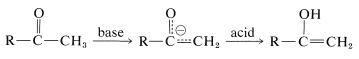## Enolization in Acid Solution

Catalysis of the enolization of 2-propanone by acids involves first, oxonium-salt formation and second, removal of an $$\alpha$$ proton with water or other proton acceptor (base):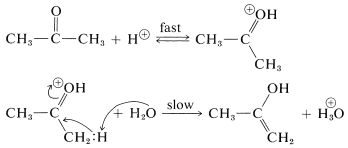This sequence differs from enolization induced by basic catalysis (as discussed in Section 17-1B) in that the enol is formed directly and not subsequent to the formation of the enolate anion. The proton addition to the carbonyl oxygen greatly facilitates proton removal from the $$\alpha$$ carbon because of the electron-attracting power of the positively charged oxygen. Nevertheless, this last step is the rate-determining for enolization in acid solution.

## Stabilities of Enols

The equilibrium position between a simple ketone and its enol usually lies far on the side of the ketone (see Table 17-2). However, there are some interesting and important exceptions to this generalization. For instance, the influence of two carbonyl groups on the enol content is very striking, as we can see from the fact that $$85\%$$ of 2,4-pentanedione is the enol form at equilibrium: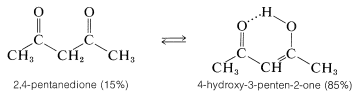Table 17-2: The Enol Content of Some Carbonyl CompoundsThe enol form of 2,4-pentanedione (and of related dicarbonyl compounds of the type) not only is stabilized by electron-delocalization, as shown in Structures $$4a$$ and $$4b$$, but by hydrogen-bonding of the acidic hydrogen between the two oxygens:Of course, such stabilization is not possible for the keto form.

An extreme example of the stabilization of an enol by electron delocalization is benzenol (phenol), which exists $$100\%$$ in the enol form. In this case the extra stability of the benzene ring is the important factor:In the succeeding sections of this chapter we will discuss several important reactions that take place by way of enols or enolate anions.

$$^1$$The important difference between 2-propanone and ethanol as acids is that the rate of establishment of equilibrium with 2-propanone or similar compounds where ionization involves breaking a $$\ce{C-H}$$ bond is very much slower than the corresponding reaction with $$\ce{O-H}$$ bonds.